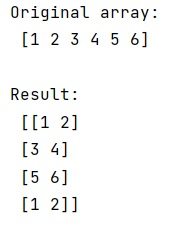# ValueError: cannot resize this array: it does not own its data in Python

Learn, how to fix NumPy's ValueError: cannot resize this array: it does not own its data in Python? By Pranit Sharma Last updated : April 06, 2023

## Reason for this ValueError

When we create an array with numpy.array() with a specific data type it results in a numpy array of the specified data type. Resizing this data is also possible by using numpy.resize() method.

However, if we apply reshape on this array and store it in another variable and then change the size of the array using resize(), it will produce the ValueError that the array does not own its data.

For example, if we have an array a and we are using reshape of a and storing it in b and applying resize on b. Here, array b is not its own array, but simply a view of a (just another way to understand the "OWNDATA" flag). In simple words, both a and b reference the same data in memory, but b is viewing a with a different shape. Calling the resize function like ndarray.resize() tries to change the array in place, as b is just a view of a, this is not permissible as from the resize definition.

## Solution for this ValueError

To fix cannot resize this array: it does not own its data error, we can use numpy.resize() rather than applying to resize function directly on the array (ndarray.resize()). NumPy will detect this issue and copy the data automatically.

Let us understand with the help of an example,

## Python code to fix "cannot resize this array: it does not own its data" ValueError

```# Import numpy
import numpy as np

# Creating an array
arr = np.array([1,2,3,4,5,6], dtype=np.uint8)

# Display original array
print("Original array:\n",arr,"\n")

# Reshaping the array
b = arr.reshape(2,3)

# Calling np.resize function
res = np.resize(b,(4,2))

# Display result
print("Result:\n",res)
```

### Output